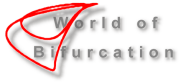## Hodgkin-Huxley Nerve Model

Original source of the model: A. L. Hodgkin, A. F. Huxley: A quantitative description of membrane current and its application to conduction and excitation in nerve. J. Physiol. 117 (1952) 500-544.

The experiments reported in Figures 1-5 concentrate on the importance of threshold values. Here it is essential whether the initial voltage U0 is below or above a threshold: the cell will fire for U0=-6, and will basically be quiet for U0=-5; compare Figures 1 and 2. Figures 4 and 5 depict in addition the dynamics of some intermediate values.

Figure 3 shows a simulation that approaches a limit cycle. Taking the applied current as bifurcation parameter,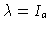, we find a Hopf bifurcation for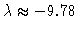with an interval of bistability of approximately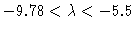.

Figure 1
(U,V1)-phase diagram of the Hodgkin-Huxley equation; two trajectories: U(0)=-5 and -6, with V1(0)=0.31, V2(0)=0.05, V3(0)=0.6 and Ia=0.

Figure 2
U(t) for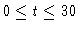; Hodgkin-Huxley equation; two trajectories: U(0)=-5 and -6, with V1(0)=0.31, V2(0)=0.05, V3(0)=0.6 and Ia=0.

Figure 3
(U,V1)-phase diagram of the Hodgkin-Huxley equation; trajectory approaching a limit cycle. U(0)=-5, V1(0)=0.31, V2(0)=0.05, V3(0)=0.6 and Ia=-10.

Figure 4
(U,V1)-phase diagram of the Hodgkin-Huxley equation; six trajectories U(0)=U0, with V1(0)=0.31, V2(0)=0.05, V3(0)=0.6 and Ia=0; U0=-5.0/-5.2/-5.4/-5.6/-5.8/-6.0

Figure 5
U(t) for; membrane potential of the Hodgkin-Huxley equation; six trajectories U(0)=U0, with V1(0)=0.31, V2(0)=0.05, V3(0)=0.6 and Ia=0; U0=-5.0/-5.2/-5.4/-5.6/-5.8/-6.0

This Example
Figure 1
Figure 2
Figure 3
Figure 4
Figure 5